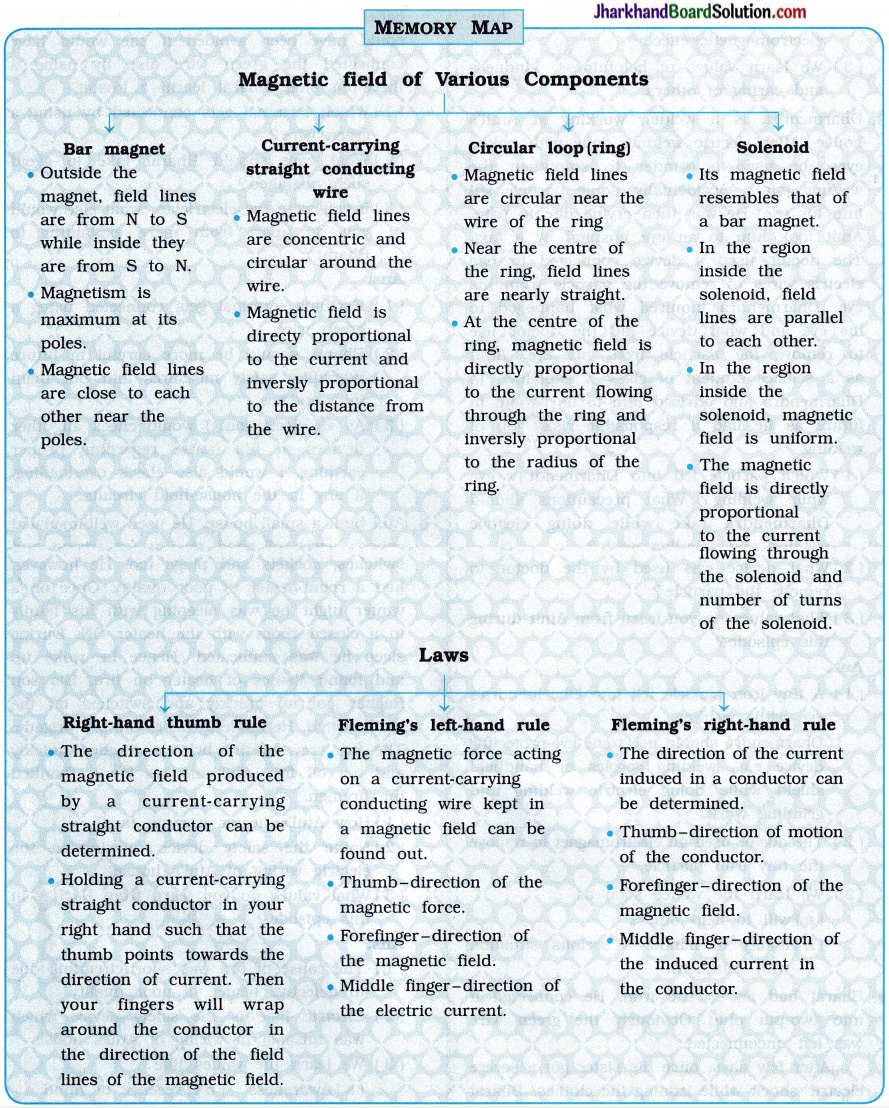# JAC Class 10 Science Important Questions Chapter 13 Magnetic Effects of Electric Current

Jharkhand Board JAC Class 10 Science Important Questions Chapter 13 Magnetic Effects of Electric Current Important Questions and Answers.

## JAC Board Class 10 Science Important Questions Chapter 13 Magnetic Effects of Electric Current

Question 1.
State at least three points of difference between the following terms / quantities :
(1) Electric motor and Electric generator

 Electric motor Electric generator 1. It converts electrical energy into mechanical energy. 1. It converts mechanical energy into electrical energy. 2. A current-carrying coil placed in a magnetic field experiences force. It works on this principle. 2. Whenever a coil is rotated in a magnetic field, an electric current is induced in the coil. It works on this principle of electromagnetic induction. 3. A current-carrying coil rotates in the magnetic field due to the equal (in magnitude) and opposite (in direction) forces acting on the parallel arms of the coil. 3. The conducting coil is made to rotate in the magnetic field by mechanical means. 4. It is used in fans, washing machines, mixers and many such appliances. 4. It is used in hospitals, shops, banks, etc. when there is a power failure.

(2) Direct Current (DC) and Alternating Current (AC)

 Direct Current (DC) Alternating Current (AC) 1. It flows only in one direction. 1. Its direction is reversed at regular intervals of time. 2. It is obtained with a cell/battery or a DC generator. 2. It is obtained with an AC generator. 3. Its production is expensive. 3. Its production is comparatively cheaper. 4. There is large loss of electric power when it is transmitted over long distances. 4. There is comparatively less loss of electric power when it is transmitted over long distances.(3) Permanent magnet and Electromagnet

 Permanent magnet Electromagnet 1. Its strength is fixed. 1. Its strength can be changed by changing the current through the coil. 2. Its polarities are fixed. 2. Its polarities can be reversed by changing the direction of the current. 3. They are usually made of alloys, e.g., Alnico Note: Alnico is an alloy of aluminium, nickel, cobalt and iron. 3. It is made by inserting a soft iron rod into a copper coil / solenoid and connecting the coil / solenoid to a battery.

Question 2.
Give scientific reasons for the following statements:
(1) It is improper to use a copper wire as a fuse wire.
The melting point of copper Is very high. Hence, it may not melt even when a current through it is more than the current rating of the circuit.
Hence, it is improper to use a copper wire as a fuse wire.

(2) We use power supply of two different current ratings at our homes.
Each electrical appliance has its rating and draws current accordingly for its function-ing. To avoid overloading and short-circuiting, two separate circuits are used in electrical wiring at home, one of 15 A for appliances with higher power ratings and another of 5 A for bulbs, tubes, fans, etc.

(3) We use AC for electric power transmission over long distances, while most of the appliances use DC.
Electric power transmission over long distances can result in a lot of loss of electrical energy as the energy gets dissipated as heat energy.

The loss of power is much less in the case of AC relative to DC. Hence, we use AC for electric power transmission over long distances.

Question 1.
Answer the following questions in one word / sentence :
(1) Name the instrument used to determine the direction of magnetic field.
Magnetic needle.

(2) At which position of a bar magnet is its magnetism maximum?
Magnetic pole

(3) Is magnetic field a scalar or a vector?
Vector

(4) What is the direction of the magnetic field inside the current-carrying circular ring as shown in the following figure?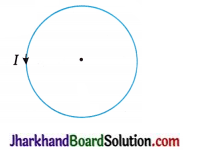Perpendicular to the plane of the paper and going into the plane of the paper

(5) As shown in the following figure, the magnetic force is acting in the downward direction on the current-carrying wire placed in the magnetic field. What is the direction of the magnetic field?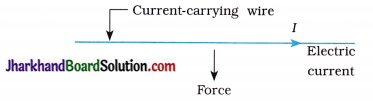Prependicular to the plane of the paper and coming out of the plane of the paper

(6) Name the scientist who first suggested that a force would act on a current-carrying wire placed in a magnetic field.
Ampere

(7) In given magnetic field, what should the direction of motion of the conductor so that the maximum induced current can be produced?
Perpendicular to the magnetic field

(8) While a magnet is moved towards a coil, will the induced current decrease or increase? Why?
Increase, because the number of magnetic field lines linked with the coil increases.(9) Which type of current can be transmitted over long distance without much loss of electrical energy?
AC

(10) Which type of connection is done for electric appliances?
Parallel

(11) Whose magnetic field resembles the magnetic field of a current-carrying solenoid?
A bar magnet

(12) How much current would be induced in a coil while a magnet and the coil both move along the same line with the same velocity?
Zero

(13) What is the other name of Right-hand thumb rule?
Maxwell’s corkscrew rule

(14) When a wire is moved up and down in a magnetic field, a current is induced in the wire. What is this phenomenon known as?
Electromagnetic induction

Question 2.
Fill in the blanks:

1. The magnetic field is ………………. in the region where the magnetic field lines are very close to each other.
2. ………………. had discovered the, magnetic effect of electric current.
3. With the help of a ………………. the presence of electric current can be known.
4. Electric motor converts ………………. energy into ………………. energy.
5. Resistance of a fuse wire is and its melting point is ……………….
6. The colour of the insulation of the earthing wire is ……………….
7. Magnetic field at the centre of a current carrying circular ring is inversly proportional to ……………….
8. In Fleming’s left-hand rule, the thumb denotes the direction of ……………….
9. The appliance with the help of which electricity can be generated is known as ……………….
10. The induced electromotive force produced in a coil is proportional to rate of change of ……………….
11. Electromagnetic induction was discovered by ……………….
12. In India, the direction of AC changes ………………. times in one second.
13. Magnetic force acting on a current-carrying wire kept in a magnetic field is in ………………. direction to the magnetic field.
14. A long wound cylindrical coil of insulated wire is called ……………….
15. Working of an electric motor is based on ………………. rule.
16. Working of an electric generator is based on ………………. rule.
17. When a coil is viewed from one end, if the current flows in an anticlockwise direction, then this end behaves like a ………………. pole.
18. When a coil is viewed from one end, if the current flows in a clockwise direction, then this end behaves like a ………………. pole.
19. In an electric motor, the split ring acts as a ……………….
20. The coil having many turns used in an electric motor or generator is called ……………….

1. Strong
2. Oersted
3. galvanometer
4. electrical, mechanical
5. high, low
6. green
8. magnetic force
9. an electric generator
10. number of magnetic field lines with time
12. 100
13. perpendicular
14. a solenoid
15. Fleming’s left-hand
16. Fleming’s right-hand
17. north
18. south
19. commutator
20. armature

Question 3.
State whether the following statements are true or false:

1. Magnetic field lines form a closed loop.
2. Magnetism is maximum at the centre of the magnet.
3. The direction of the magnetic field produced by the current-carrying conducting wire is determined by Fleming’s left-hand rule.
4. While going away from the magnet, magnetic field becomes strong.
5. At every point inside the region of a long solenoid, magnetic field is uniform.
6. Electrical generator converts electrical energy into mechanical energy.
7. In India, the frequency of AC is 50 Hz.
8. All electrical appliances in a house are connected in series.
9. The insulated cover on the neutral wire is black in colour.
10. There is a link between electric current and magnetic field.
11. Magnetic field lines intersect each other.
12. Magnitude of the current induced in a circular loop depends on the speed of the magnet moving towards it.
13. The direction of DC remains constant in time.
14. Electromagnet consists of a current-carrying solenoid with Alnico rod (an alloy) put in it.
15. Overloading is caused by connecting too many appliances in a single socket and using them at the same time.
16. A stationary charge is surrounded by a magnetic field.
17. It is safe to touch a live wire.

1. True
2. False
3. False
4. False
5. True
6. False
7. True
8. False
9. True
10. True
11. False
12. True
13. True
14. False
15. True
16. False
17. False

Question 4.
Match the following :
(1)

 Section ‘A’ Section ‘B’ 1. Oersted p. Force would act on a current-carrying conducting wire when it is placed in a magnetic field. 2. Faraday q. The rule to find the direction of the force acting on a current-carrying conductor placed in a magnetic field. 3. Ampere r. When an electric current is passed through a wire, a magnetic field is produced. 4. Fleming s. Induced current is produced in the coil, while moving a magnet towards the stationary coil.

(1 – r), (2 – s), (3 – p), (4 – q).

(2)

 Section ‘A’ Section ‘B’ 1. Right-hand thumb rule p. Gives the direction of the current induced in a conductor. 2. Fleming’s right-hand rule q. Gives the direction of the force acting on a current-carrying conductor placed in a magnetic field. 3. Flemintg’s left-hand rule r. Gives the direction of the magnetic field around a current-carrying straight conductor.

(1 – r), (2 – p), (3 – q)

(3)

 Section ‘A’ Section ‘B’ 1. Current-carrying solenoid p. Is used to lift heavy objects made of iron. 2. Fuse q. Converts mechanical energy into electrical energy. 3. Electromagnet r. Behaves like a bar magnet. 4. Electric motor s. Converts electrical energy into mechanical energy. 5. Electric generator t. Is used to prevent damage to the appliances and the circuit due to overloading.

(1 – r), (2 – t), (3 – p), (4 – s), (5 – q).Question 5.
Choose the correct option from those given below each question:
1. The direction of the magnetic field lines in a region outside a bar magnet is …
A. from N-pole to S-pole of the magnet.
B. from S-pole to N-pole of the magnet.
C. coming out from both the poles of the magnet.
D. entering into both the poles of the magnet.
A. from N-pole to S-pole of the magnet.

2. Which of the following statements is false?
A. The direction of the magnetic field line in a region outside a bar magnet is from N to S.
B. In the region where the magnetic field lines are at a close distance from each other, there is a strong magnetic field.
C. The magnetic field lines form closed loops.
D. The magnetic field lines can cross each other.
D. The magnetic field lines can cross each other.

3. By which instrument can the presence of magnetic field be determined?
A. Voltmeter
B. Ammeter
C. Galvanometer
D. Magnetic needle
D. Magnetic needle

4. Who discovered the magnetic effect of an electric current?
B. Oersted
C. Volta
D. Ampere
B. Oersted

5. With the help of which law/rule can the direction of a magnetic field be decided?
B. Fleming’s right-hand rule
C. Right-hand thumb rule
D. Fleming’s left-hand rule
C. Right-hand thumb rule

6. According to the right-hand thumb rule, whose direction is indicated by the thumb?
A. Electric current
B. Magnetic field
C. Magnetic force
D. Motion of a conductor
A. Electric current

7. The magnetic field produced by a straight conducting wire on passing the current through it is …
A. in the direction of the current.
B. in the direction opposite to that of the current.
C. circular around the wire.
D. in the direction parallel to the wire.
C. circular around the wire.

8. What is the shape of the field line of a magnetic field passing through the centre of a current-carrying circular ring?
A. Circular
B. Straight line
C. Ellipse
D. Magnetic field is zero at the centre of the ring
B. Straight line

9. Whose magnetic field is like the magnetic field of a bar magnet?
A. A current-carrying wire
B. A current-carrying ring
C. A current-carrying solenoid
D. A horse-shoe magnet
C. A current-carrying solenoid

10. Who discovered electromagnetic induction?
B. Oersted
C. Ampere
D. Volta11. What is the direction of the magnetic force acting on a current-carrying wire placed in a magnetic field?
A. Along the magnetic field
B. Along the electric current
C. Perpendicular to the magnetic field
D. Opposite to that of the magnetic field
C. Perpendicular to the magnetic field

12. How is a current-carrying wire placed in a magnetic field so that a magnetic field does not act on it?
A. Parallel to the magnetic field
B. Perpendicular to the magnetic field
C. At an angle of 45° with magnetic field
D. At an angle of 120° with magnetic field
A. Parallel to the magnetic field

13. State in which of the following cases, the induced current in the loop will not be obtained.
A. The loop is moved towards the magnet.
B. The magnet is moved towards the loop.
C. The loop and the magnet are moved in the opposite directions with the same speed.
D. The loop and the magnet are moved in the same direction with the same speed.
C. The loop and the magnet are moved in the opposite directions with the same speed.

14. Which instrument is used to convert electrical energy into mechanical energy?
A. Electric generator
B. Electric motor
C. Electric iron
D. Electric oven
B. Electric motor

15. On which principle does the electric generator work?
A. Electrical energy is converted into mechanical energy.
B. Electrical energy is converted into heat.
C. Mechanical energy is converted into electrical energy.
D. Electrical energy is converted into light.
C. Mechanical energy is converted into electrical energy.

16. The magnitude of the AC voltage used in India is ……………. and its frequency is …………….
A. 110 V 60 Hz
B. 110 V, 50 Hz
C. 220 V, 50 Hz
D. 220 V, 60 Hz
C. 220 V, 50 Hz

17. A wire with ……………. insulation is used for earthing.
A. red
B. black
C. green
D. white
C. green

18. Which type of current is obtained from a battery?
A. DC
B. AC
C. AC and DC
D. Depends upon the type of the battery
A. DC

19. Which instrument is used to know the presence of an electric current?
A. Fuse
B. Galvanometer
C. Voltmeter
D. Magnetic needle
B. Galvanometer

20. A fuse wire is a/an …………….
A. conductor having low melting point
B. insulator having low melting point
C. semiconductor having low melting point
D. conductor having high melting point
A. conductor having low melting point

21 is used to find the direction of a current induced in a circuit.
A. Fleming’s left-hand rule
B. Fleming’s right-hand rule
C. Right-hand thumb rule
D. Ampere’s rule
B. Fleming’s right-hand rule

22. How many times in one second does an AC with frequency 50 Hz change its direction?
A. 25
B. 50
C. 100
D. 200
C. 100

23. At the centre of which of the following four circular rings is the magnetic field maximum while passing equal current through each of them?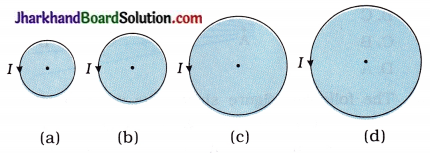A. (a)
B. (b)
C. (c)
D. (d)
A. (a)

24. Inside a bar magnet the direction of the magnetic field lines is …………….
A. from N-pole to S-pole of the magnet
B. from S-pole to N-pole of the magnet
C. coming out from both the poles of the magnet
D. entering into both the poles of the magnet
B. from S-pole to N-pole of the magnet25. When the north poles of two different magnets are placed near each other, then the force and when the north pole and the south pole are placed near each other the force of ……………. results.
A. attraction and attraction
B. attraction and repulsion
C. repulsion and attraction
D. repulsion and repulsion
C. repulsion and attraction

26. Which figure, out of the following, shows the magnetic field lines of a bar magnet?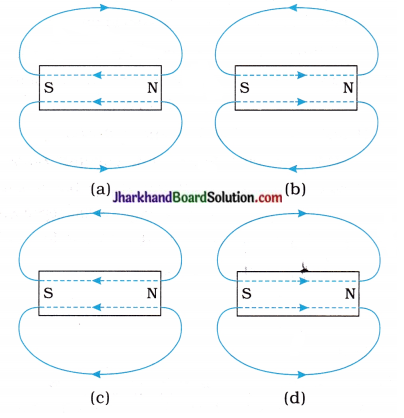A. (a)
B. (b)
C. (c)
D. (d)
B. (b)

27. The following figure shows the magnetic field of a magnet. At which point is the intensity of the magnetic field more?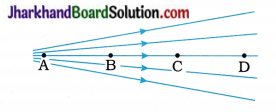A. D
B. C
C. B
D. A
D. A

28. The following figure shows the magnetic field between two magnets. Which magnetic poles are there at points A and B respectively?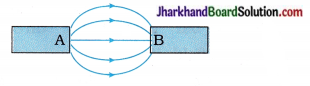A. South pole, north pole
B. North pole, south pole
C. North pole, north pole
D. South pole, south pole
B. North pole, south pole

29. On what factor does the direction of the magnetic field produced by a current-carrying straight wire depend?
A. The magnitude of the electric current
B. The direction of the electric current
C. The length of the straight wire
D. The material of the straight wire
B. The direction of the electric current

30. The magnetic field at the centre of a current carrying circular loop is ……………. to the current and ……………. to the loop’s radius.
A. inversely proportional, directiy proportional
B. directiy proportional, directiy proportional
C. directly proportional, inversely proportional
D. inversely proportional, inversely proportional
C. directly proportional, inversely proportional

31. As we move away from a current-carrying straight wire, the magnetic field of wire varies as ……………. (Where, r is the distance between a given point and the wire.)
A. r²
B. $$\frac { 1 }{ r }$$
C. $$\frac { 1 }{ r² }$$
D. r
B. $$\frac { 1 }{ r }$$

32. Out of the following, which statement is wrong for a current-carrying solenoid?
A. The magnetic field inside the solenoid is the same at each point.
B. Placing an iron object inside the solenoid increases the magnetic field.
C. The pattern of the magnetic field lines of the solenoid is different from that of the magnetic field lines of a bar magnet.
D. On reversing the direction of the current through the solenoid, the positions of N-pole and S-pole of the solenoid also get reversed.
C. The pattern of the magnetic field lines of the solenoid is different from that of the magnetic field lines of a bar magnet.

33. When an iron piece is introduced inside a current-carrying solenoid the magnetic field of the solenoid …………….
A. increases
B. decreases
C. first increases then decreases
D. does not change
A. increases34. The magnetic force acting on a current-carrying wire placed in a magnetic field is directiy. proportional to …………….
A. the electric current
B. the magnetic field
C. the length of the wire in the magnetic field
D. all the given
D. all the given

35. When electric current I flows through a straight wire of length l placed in a magnetic field, a magnetic force of 1 N acts on it. If magnitude of the electric current is halved and the magnetic field is doubled, what will be the magnetic force acting on the wire?
A. 0.5 N
B. 1 N
C. 2N
D. 4 N
B. 1 N

36. As shown in the following figure, a current-carrying coil ABCD is placed in a magnetic field. Which portion of the coil does not experience any magnetic force?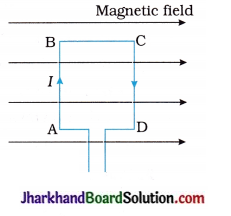A. Portion AB
B. Portion BC
C. Portion CD
D. Both A and B
B. Portion BC

37. What happens when a bar magnet is quickly moved towards a coil?
A. The resistance of the coil increases.
B. The magnetic field linked with the coil decreases.
C. The magnetic field linked with the coil increases.
D. The coil moves towards the magnet.
C. The magnetic field linked with the coil increases.

38. In which of the following cases will the electric current induced in the loop be maximum?
A. The magnetic field linked with the loop changes rapidly.
B. The magnetic field linked with the loop changes slowly.
C. The magnetic field linked with the loop remains constant.
D. The loop is placed in an electric field.
A. The magnetic field linked with the loop changes rapidly.

39. What is the frequency of 220 V DC voltage?
A. 50 Hz
B. 60 Hz
C. 220 Hz
D. Zero
D. Zero

40. What is the colour of insulation on the neutral wire coming from the electric board?
A. Red
B. Black
C. Green
D. White
B. Black

41. What is the red coloured wire coming from the electric main board called?
A. The earthing wire
B. The neutral wire
C. The live wire
D. The fuse wire
C. The live wire

42. On which effect of electric current does the fuse work?
A. Magnetic effect of electric current
B. Chemical effect of electric current
C. Heating effect of electric current
D. Electric effect of electric current
C. Heating effect of electric current

43. At the time of short circuit, the electric current ……………….
A. suddenly decreases
B. suddenly increases
C. instantaneously becomes zero
D. does not change
B. suddenly increases

44. The direction of the current in the coil of an electric motor changes per ………………. rotation.
A. two
B. one
C. half
D. one-fourth
C. half

45. In the following figure, a magnetic needle is placed at different positions near a bar magnet. Which position of the magnetic needle represents the correct direction of the magnetic field?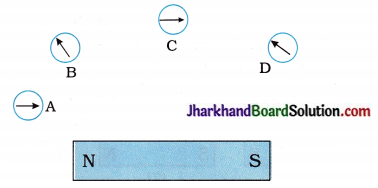A. A
B. B
C. C
D. D
C. C

46. On which metallic piece is the conducting wire wound to prepare an electromagnet?
A. Copper
B. Soft iron
C. Aluminium
D. Nichrome
B. Soft iron47. To double the voltage generated in a generator, ……………….
A. the rotational speed of the coil should be halvened.
B. the number of turns of the coil should be doubled.
C. the cross-sectional area of the coil should be halvened.
D. none of the given
B. the number of turns of the coil should be doubled.

48. In the following figure, magnetic field of different current-carrying wires is shown. Which figure represents the correct direction of the magnetic field?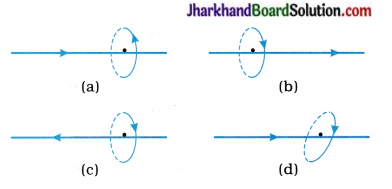A. Position A
B. Position B
C. Position C
D. Position D
B. Position B

49. A current-carrying conducting wire kept in a magnetic field should experience a force. This was suggested by ……………….
A. Oersted
C. Ampere
D. Ohm
C. Ampere

50. The magnitude of the induced current in electromagnetic induction depends on ……………….
A. the motion of the coil only
B. the motion of the magnet only
C. the relative speed of the coil and magnet
D. the deflection of the pointer in the galvanometer
C. the relative speed of the coil and magnet

51. In Fleming’s left-hand rule, ………………. denotes the direction of the magnetic force.
A. the thumb
B. the forefinger
C. the middle finger
D. the palm
A. the thumb

52. In the following figure, three bar magnets are shown. Magnets (1) and (3) are fixed. In which direction will the magnet (2) move?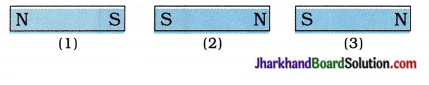A. Towards magnet (1)
B. Towards magnet (3)
C. Upward
D. Magnet (2) will remain at rest
B. Towards magnet (3)

53. To find the direction of the magnetic field produced by a current-carrying wire, a magnetic needle is placed at different positions as shown in the following figure. Which position of the magnetic needle corresponds to the correct direction of the magnetic field?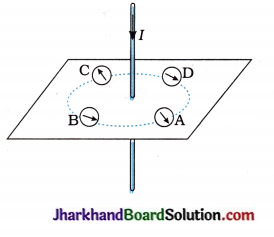A. Position A
B. Position B
C. Position C
D. Position D
D. Position D

54. In the following figure, current-carrying loop ABCD is placed in a uniform magnetic field. The magnetic forces acting on wires AB and CD are in ………………. and ………………. direction respectively.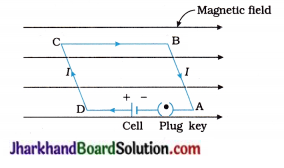A. Downward, upward
B. Downward, downward
C. Upward, upward
D. Upward, downward
D. Upward, downward

Question 6.
Answer the following questions in very- short as directed (miscellaneous):
(1) Name the physical qualities which are indicated by the direction of the thumb and forefinger in Fleming’s right-hand rule.
The thumb denotes direction of motion of the conductor and the forefinger indicates the direction of the magnetic field.

( 2) State the effect on the strength of the magnetic field produced at a point near a straight conductor if the electric current flowing through it increases.
The strength of the magnetic field increases.

(3) Mention the angle between a current carrying straight conductor and magnetic field for which the force experienced by the conductor placed in a magnetic field is maximum.
90°

(4) List two sources of magnetic fields.
Magnet, moving charges in space, electric current through conductor.

(5) There are two wires. One of them carries a current. How will you find out which one carries a current?
Bring a magnetic needle near the wire. The current-carrying wire will produce a deflection in the needle whereas the wire without a current will not.

(6) How will the magnetic field intensity B at the centre of a circular coil carrying current change, if the current through the coil is doubled and the radius of the coil is halved?
The magnetic field at the centre of the coil, B ∝ $$\frac { I }{ R }$$.
When current I is doubled and radius R is halved, the magnetic field at the centre of the coil becomes four times the original / initial magnetic field.

(7) PQ is a current-carrying long straight conductor in the plane of the paper as shown in the following figure. A and B are two points at distances r1 and r2 from it.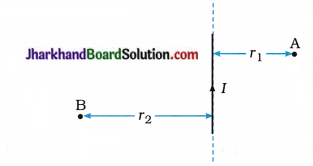If r2 > r1 at which point will the strength of the magnetic field be greater? Justify your answer.
The magnetic field at a point near the current-carrying straight conductor is B ∝ $$\frac { 1 }{ r }$$.
Hence, at point A B1 ∝ $$\frac{1}{r_1}$$ and at point B B2 ∝ $$\frac{1}{r_2}$$
As r1 < r2, B1 > B2.

(8) PQ is a current- carrying long straight conductor in the plane of the paper as shown in the following figure. A and B are two points at distances r1 and r2 from it.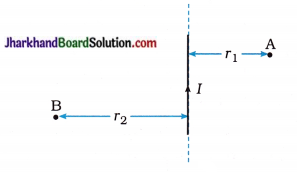Mention the direction of the magnetic field produced by it at points A and B.
Using the Right-hand thumb rule and S properties of magnetic fields lines we find that at point A magnetic field intensity would be perpendicular to the plane of paper in the inward direction (x) going into the plane and at point B it would be perpendicular to the plane of paper in the outward direction (.) [coming out of the plane.]

(9) In the experiment to show that a current-carrying conductor (rod) when placed in a uniform? magnetic field experiences a force. What will happen if you interchange the terminals of the battery connected in the circuit?
The conductor (rod) will be deflected in the opposite direction as the direction of the current is reversed.

(10) A compass needle is placed near a current-carrying straight wire. State your observation when the current in the wire is increased. Give reason.
The deflection of the compass needle increase.
Reason: Magnetic field strength produced s near a current-carrying straight wire is directly proportional to the current.

(11) The magnetic field lines of two bar s magnets A and B are shown below: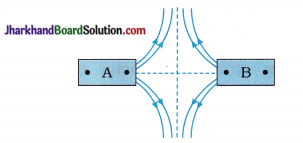Name the poles of the magnets facing each other.
North poles

(12) Name any two sources of direct current.
Electric cell and DC generator.

(13) Name the device used to prevent damage to the electrical appliances and domestic circuit due to overloading or short-circuiting.
Electric fuse(14) State value of potential difference between l the live wire and neutral wire of power supply in our country.
220 V

(15) State one difference between the wires used in the element of an electric heater and in a fuse.
The melting point of the material of the wire used in a heater element is high while a fuse wire has low melting point.

(16) In a domestic electric circuit-wiring, with which wire do we connect a fuse?
The live wire

(17) Mention two ways to increase the strength of the magnetic field of a current-carrying solenoid.
Two ways to increase the strength of the magnetic field of a current-carrying solenoid :

• Increasing the number of turns of the solenoid.
• Increasing the current through the solenoid.

(18) Give the significance of the electric meter in a domestic circuit.
Electric meter is used to record the consumption of electrical energy in kWh [unit] (commercial unit of electric energy) in the domestic circuit.

(19) A domestic circuit has a line of 5 A rating. How many lamps of ratings 40 W, 220 V can be run simultaneously on this line safely?
The current rating of one lamp,
I = $$\frac { P }{ V }$$ = $$\frac { 40 W }{ 220 V }$$ = $$\frac { 2 }{ 11 }$$ A
Thus, current of $$\frac { 2 }{ 11 }$$A is required by one lamp to glow completely.
Now, the current rating (i.e., Maximum current in the line) = 5 A.
∴ The number of lamps that can be run simultaneously on this line safely.
= $$\frac{\text { current rating of the line }}{\text { current rating of the lamp }}$$
= $$\frac{5 \mathrm{~A}}{\frac{2}{11} \mathrm{~A}}$$
= $$\frac { 55 }{ 2 }$$
= 27.5
≈ 27

(20) Give any two uses of an electromagnet.

1. In electric bells
2. For separating magnetic substances such as iron and other substances having magnetic property from a metallic scrap.
3. In hospitals, doctors use it to remove particles of iron or steel from a patient’s eye.

(21) A constant current I flows in a horizontal wire in the plane of paper from east to west as shown in the following figure :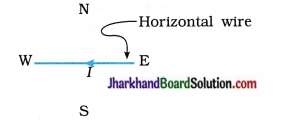At which point will the direction of the magnetic field be from north to south?
According to the Right-hand thumb rule, when a current-carrying wire is held in the right hand, with the stretched thumb from east to west in the direction of the current, the fingers wrapped around the wire will be from north to south at the point lying directly below the wire. Hence, the magnetic field at that point would be from north to south.

(22) How do you magnetise a piece of magnetic material?
A piece of magnetic material can be magnetised by keeping it inside a current-carrying solenoid.

(23) How does the current in a domestic circuit differ from that used to run a clock?
The current in a domestic circuit is an alternating current (AC), while the current used to run a clock is a direct current (DC).

(24) State what would happen to the direction of rotation of the coil of an electric motor, if the direction of the current flowing through the coil is reversed.
The direction of rotation of the coil would be reversed.

(25) State the use of Maxwell’s corckscrew rule.
Maxwell’s corkscrew rule is used to find the magnetic polarities of a current-carrying coil or a solenoid.

(26) What type of dynamo (a machine for changing other power into electric power or a machine to produce electric current) is used in (i) a bicycle (ii) industry?
In a bicycle DC dynamo is used while in industry AC dynamo is used.

(27) Give one reason for not using a series arrangement for domestic circuits.
If one of the appliances does not work and consequently the circuit becomes incomplete other appliances in the domestic circuit will stop working.

(28) What does the following symbol indicate / represent?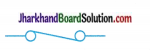Electric fuse

(29) What is the function of the brushes in an AC generator?
In 311 AC generator brushes are used to transfer current from the simple coil / armature of the generator to the load in (extermal) circuit.

(30) What determines the frequency of AC produced by a generator?
The frequency of AC produced by a generator is determined by the rotation of the coil and is equal to the frequency of rotation of the coil.

(31) What is the role of each of the three pins in a power plug?
The thick pin at the top of a power plug is for earthing. The live pin is on the left and the neutral pin is on the right of the power plug.

(32) State two serious hazards of electricity.

1. If a person accidently touches the live wire, he gets a severe shock which may prove fatal.

(33) Name an instrument used in navigation.
Magnetic compass needle

(34) What is the effect on the resistance in the circuit at the time of short-circuit?
At the time of short-circuit, the resistance in the circuit becomes very small (nearly zero).

(35) Name the current-carrying conductor, whose magnetic field is represented by the following diagram :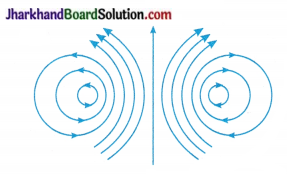A Circular coil.

(36) What is a commutator?
A device that reverses the direction of s flow of current through the circuit of an electric? motor is called a commutator.

(37) In an electric motor, which of the s following remains fixed and which rotates with the coil?
(i) Commutator
(ii) Brush
(i) Commutator rotates with the coil.
(ii) Brush remains fixed.

(38) What change should be made in an AC generator, so that it may become a DC generator?
If we replace rings R1 and R2 of an AC generator by a split ring type commutator, then it will become a DC generator.

(39) If fuses of 250 mA, 500 mA, 1 A, 5 A and 10 A were available, which one would be the most suitable for protecting an amplifier rated as 240 V, 180 W?
1 A
Because, the current rating of an amplifier is
I = $$\frac { P }{ V }$$ = $$\frac { 180 W }{ 240 V }$$ = $$\frac { 3 }{ 4 }$$ A = 0.75 A
Hence, the fuse of 1 A rating is most suitable compared to other given fuses for safety purpose.

(40) What is a galvanometer?
A galvanometer is an instrument used to detect the presence of an electric current in a circuit.

(41) What are the two most commonly used domestic circuits?

1. 5 A for low power rating appliances.
2. 15 A for high power rating appliances.

(42) What do you mean by earthing?
Earthing means connecting the metal case of an electrical appliance to the earth (at zero potential) by means of a metal wire called the ‘earth wire’.

Question 1.
Shalini and his brother Amey observed a water-pump working. Shalini inquired how an electric motor works. Amey collected different parts of an electric motor e.g., field – magnets, cabon – brushes, armature, battery, switch, connecting wire, split-rings, etc. and showed them to Shalini. Amey then explained how an armature could rotate.

1. State the principle how an electric motor works.
2. Is an electric motor needed for water-pump to work?
3. What values do you learn from Amey’s activities?

1. When a current-carrying coil is placed in a magnetic field, it experiences a force. The direction of this force is found by using the Fleming’s left-hand rule.
2. Yes. An electric motor is needed for water-pump to work.
3. Aptitude for practical demonstration and enthusiasm to learn.

Question 2.
Jay’s friend Dev was playing and that too with a magnet. Jay asked him not to play with a magnet. Even then Dev continued playing and brought the magnet very near to the TV screen. That TV then got damaged and there was a dark patch on that area of TV due to effect of magnetism. Jay informed all of his friends to be aware of such things in future.

1. How was TV damaged to get dark patch, when the magnet was brought closer?
2. Name any two domestic devices having electromagnetic effect.
3. What values do you learn from Jay?

1. TV set has an electromagnet installed in it. When a magnet is brought closer to the TV screen, the two magnetic fields interfered with each other and spoiled the functioning of the TV.
2. Mobile phones and CD players have electromagnetic effect.
3. We learn values of helpfulness, kindness and caring of others.

Question 3.
Dharmendra is a welder, working at Amit’s house. After electric welding done with naked eyes, he used a grinder to smoothen the welding joints. Accidentally, some particle fell into his eye. He was then crying due to pain. Amit took him to an eye hospital by a taxi. The doctor used a device connected to two electric wires to remove the particle from his eye.

Dharmendra inquired what happened to his eye and what device did the doctor use to remove the particle from his eye. Amit as a science student of class X explained to Dharmendra and advised him to be careful in future as he had a responsible risky job of welding.

1. Which particle fell into Dharmendra’s eye while welding? What precautions should Dharmendra take while doing electric welding and grinding work?
2. What device was used by the doctor to remove the particle?
3. What values do you learn from Amit during this episode?

1. A tiny iron particle fell into Dharmendra’s eye while welding. Dharmendra should use some eye-protecting device e.g., welding goggles or handheld shield while doing electric welding and grinding work.
2. The doctor used an electromagnet to remove the tiny iron particle.
3. We learn the values such as (a) will to help others (b) ability to handle a serious situation with calmness.Question 4.
Bharat had an electric iron. He connected it into two-pin plug. Obviously the green wire was left unconnected. After few days, once his sister got a severe electric shock while ironing the clothes. Bharat called the electrician. He told that this situation could have been avoided if she would have connected the green wire also by using a three-pin plug. Bharat learnt a lesson.

1. Which terminal gets connected by using a green wire?
2. What care should Bharat take to avoid such mistakes?
3. If you were the electrician, what else would you do apart from mere explaining to Bharat?

1. Earthing terminal gets connected using a green wire.
2. Bharat should be more careful in future regarding safety measures and put them into practice.
3. As an electrician, I would check all other devices at his house regarding proper earthing. I would also check overloading, if any in the house-hold circuits.

Question 5.
Atul built a small house. He used well-insulated copper wires of good quality for wiring and switches, sockets and plugs too. He however, had a room-heater of poor quality. Once on a winter night .he was sleeping with his family in a closed room with the heater ON.

During sleep he was suffocated. Hence he woke up and found flames of heater on fire. His son Raghav got up quickly and switched off the main switch. He made a call to the fire brigade. The fire crew of fire brigade reached quickly and put off fire. Atul and his family thanked the firemen.

1. How Atul’s heater was on fire?
2. Name the safety device missing in the electric wiring of Atul’s house.
3. What values do you learn from Raghav in this episode?